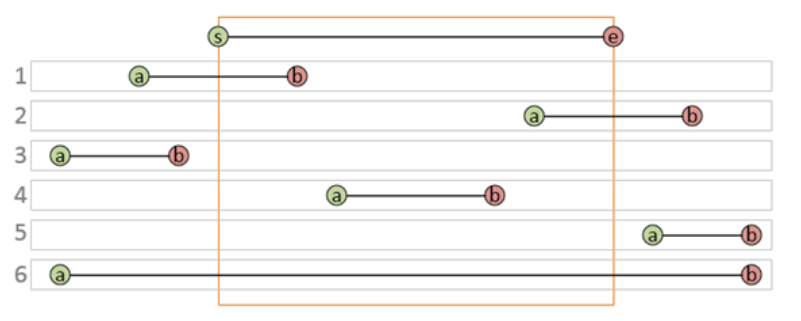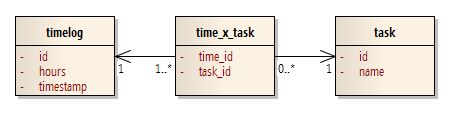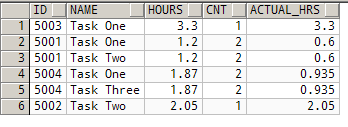Areas of Interest, as counted by my cat

# Category: SQL Syntax

Consider an Event table, with columns A and B defining the start and end of the period of time over which the event occurred.
Consider also a random period of time defined from S to E.
Now imagine that you want to select all event rows that overlap that specific period of time.

By overlap, we mean that case 1,2,4, and 6 should be included, but 3 and 5 should not:The first solution that might occur to us is this one:

`select * from Event where B between S and E  or  A between S and E`
At first glance it looks clear and elegant, but unfortunately it doesn’t handle case #6.
Somewhat less intuitive but still elegant and also complete is:

`select * from Eventwhere B >= S and A <= E`

Say we want create a generated list of values – sequential dates, for example – as a set of rows. We can do this using recursive common table expressions.

Here’s an example of a common table expression to generate a set of dates:

`with dates as(   select date '2012-01-01' as DATE_VALUE from dual   union all   select date '2012-01-02' as DATE_VALUE from dual   union all   select date '2012-01-03' as DATE_VALUE from dual)select * from dates;`

Using this technique, we could create a set of rows with sequential date values… but it is not very flexible. Ideally, we want a technique that expresses the intent algorithmically.

In 11g R2 and later, we can use a reference to the outer table recursively from inside the definition! This sounds crazy but it works:

`with dates as(   select date '2012-01-01' as DATE_VALUE from dual   union all   select d.DATE_VALUE + interval '1' day as DATE_VALUE   from dates d   where d.DATE_VALUE < date '2013-01-01')select * from dates;`

In this example, we are UNIONING the first row with a sub-select against itself, adding a new row that increments the date value by 1.

The first time I tried this technique, I got the following error:

ORA-32039: recursive WITH clause must have column alias list

Some research on the web gave me a page that explained what the problem was. The error message is actually very clear: we need to add a list of columns at the top of the WITH <alias> clause:

`with dates ( DATE_VALUE ) as(   select date '2012-01-01' as DATE_VALUE from dual   union all   select d.DATE_VALUE + interval '1' day as DATE_VALUE   from dates d   where d.DATE_VALUE < date '2013-01-01')select * from dates;`

And now, it just works:

`SQL>DATE_VALUE-----------2012-01-012012-01-022012-01-032012-01-042012-01-052012-01-062012-01-072012-01-082012-01-092012-01-102012-01-11:`

Aside: Here’s how to do the same query in PostgreSQL:

`with recursive dates ( DATE_VALUE ) as(   select date '2012-01-01' as DATE_VALUE    union all   select cast(d.DATE_VALUE + interval '1' day as date) as DATE_VALUE   from dates d   where d.DATE_VALUE < date '2013-01-01')select * from dates;   `

The nice thing about this technique is that we can control the end date, and the increment size, by variables:

`define _increment = '5';define _start     = date '2012-01-01';define _end       = date '2012-02-01'; with dates ( DATE_VALUE ) as(   select &_start as DATE_VALUE from dual   union all   select d.DATE_VALUE + interval '&_increment' day as DATE_VALUE   from dates d   where d.DATE_VALUE < &_end)select * from dates;`

This gives us a list of dates 5 days apart.

### Using this technique in a PL/SQL block

If you’re in a PL/SQL block, trying to generate a list dynamically based on parameter values, the code will need to look slightly different:

`declare   p_start_time date;   p_interval_days integer;   p_end_date date;   --   l_interval interval day to second;begin   l_interval := numtodsinterval( p_interval_days, 'day' );   insert into My_List_Of_Dates   with   dates ( DATE_VALUE ) as   (      select         p_start_time as DATE_VALUE      from dual      union all      select         DATE_VALUE + l_interval as DATE_VALUE      from dates d      where d.END_TIME < p_end_date   )   select DATE_VALUE from dates;end;`

Sometimes I forget how to do simple things, like splitting a string into sections based on a delimiter.

Here’s a nice way to test your code on a range of input values, and see the interim results, in one hit:

`with myobject as (   -- put in a range of test values:   select 'dbo.MyFunction' as NAME   union   select 'setup.ins_Contact'   union   select 'MyOtherFunction'),more2 as (   -- calculate interesting attributes:   select      NAME,      len( NAME )            as LENGTH_,      charindex( '.', NAME ) as POSITION   from myobject)-- perform the test:select    NAME, POSITION, LENGTH_,   case when POSITION=0 then 'dbo'                        else substring( NAME, 1, POSITION-1 )                         end as SCHEMA_,   substring( NAME, POSITION+1 , (LENGTH_ - POSITION ) ) as OBJECT_from more2;;`

I found that I needed to convert some raw column names (i.e. THIS_IS_MY_COLUMN) into a human-readable “Proper” or “Title” case form, e.g. This Is My Column.

Scouring the web, I didn’t find out I could steal, so here’s my implementation:

`   function Proper(      p_string varchar2   ) return varchar2   is      l_string varchar2(200);      l_proper varchar2(200);      begin      l_proper := '';      -- change any underscores to spaces:      l_string := replace(lower(p_string), '_',' ' );      for i in 1..length(l_string)      loop         -- obviously the first character is UPPER:         if i =1 then            l_proper := l_proper || upper( substr( l_string, i, 1 ) );         else         -- if the character is preceded by a space, UPPER it:            if substr( l_string, i-1, 1 ) = ' ' then               l_proper := l_proper || upper( substr( l_string, i, 1 ) );            else               l_proper := l_proper || substr( l_string, i, 1 );            end if;                     end if;         end loop;      return l_proper;   end;`
` `

Say you have a data model that looks like this:In other words, time entries can be tagged against multiple tasks. (I know this seems wrong, but trust me, it made sense in the context of the system.)

The problem we had was that the complicated query behind the report of “total hours by task” was counting the hours twice or more, for any entry tagged to more than one task.

[code:c#]select T.NAME, sum(L.HOURS)
join timelog L on L.ID=X.TIME_ID
group by T.NAME; [/code]

After thinking about how I could alter the query so that each timelog.HOURS was included only once in the SUM(), it occurred to me that it might be better to just distribute the hours across the tasks:

[code:c#]select L.ID, T.NAME, L.HOURS, L2.cnt, (L.HOURS/L2.CNT) as ACTUAL_HRS
join timelog L on L.ID=X.TIME_ID
join (select count(1) as CNT, TIME_ID